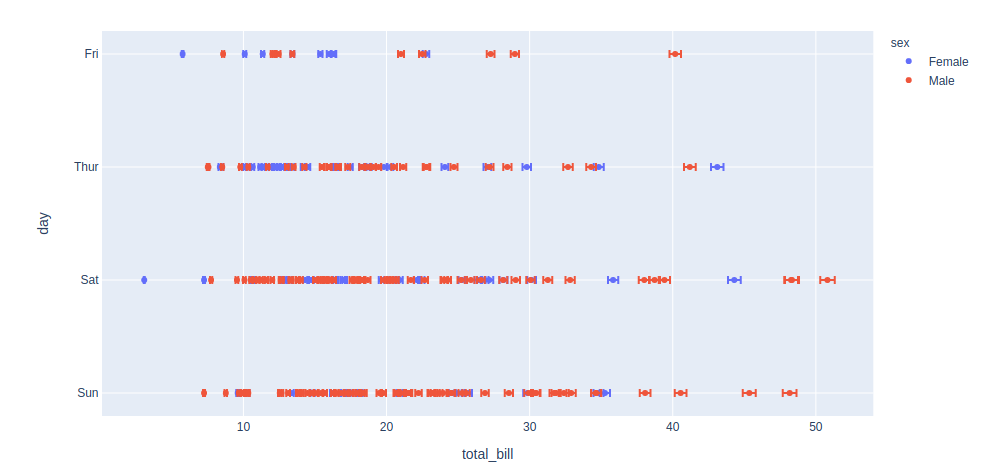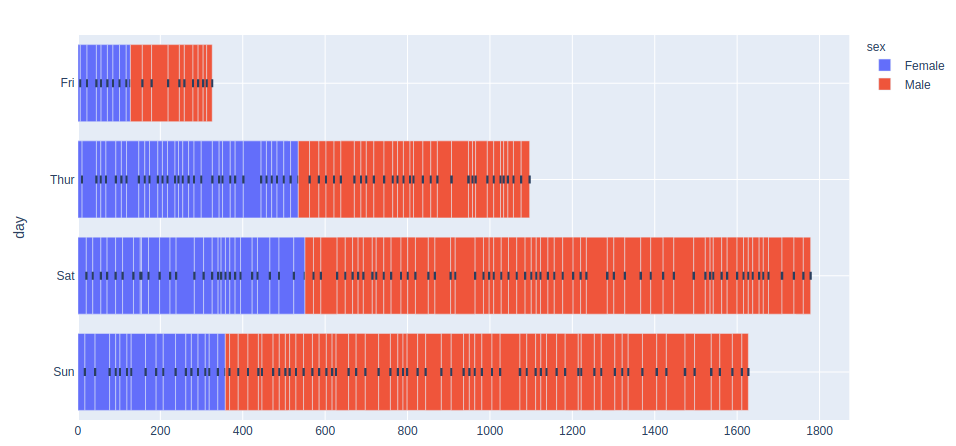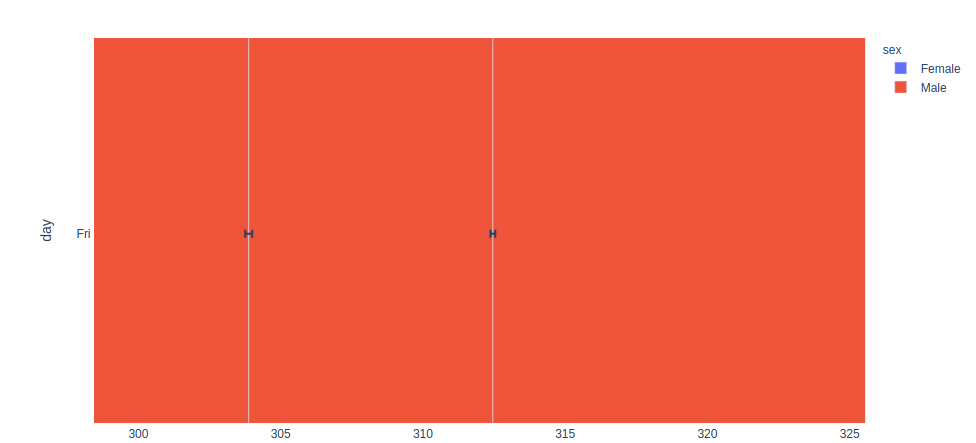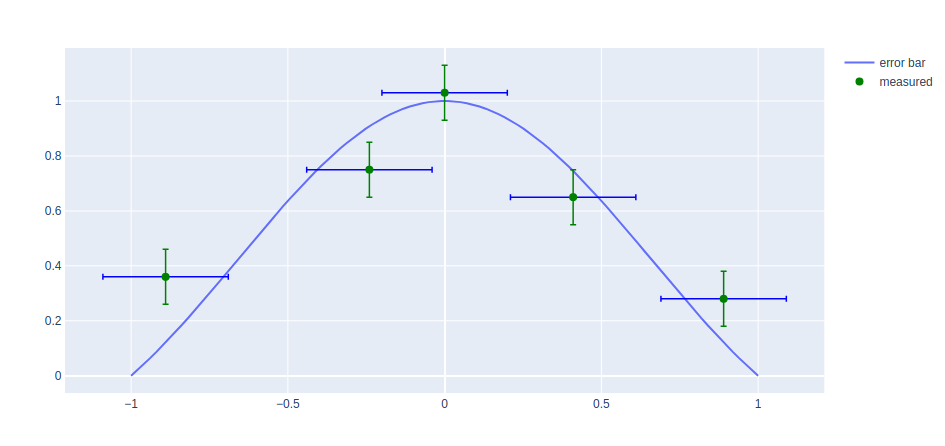Related Articles
Create Error Bars in Plotly – Python
• Last Updated : 05 Sep, 2020

Plotly is a Python library that is used to design graphs, especially interactive graphs. It can plot various graphs and charts like histogram, barplot, boxplot, spreadplot, and many more. It is mainly used in data analysis as well as financial analysis. plotly is an interactive visualization library.

## Error Bars in Plotly

For functions representing 2D data points such as px.scatter, px.line, px.bar, etc., error bars are given as a column name which is the value of the error_x (for the error on x position) and error_y (for the error on y position). Error bars are the graphical presentation alternation of data and used on graphs to imply the error or uncertainty in a reported capacity.

Example 1:

## Python3

 `import` `plotly.express as px`` ` ` ` `df ``=` `px.data.tips()``df[``"error"``] ``=` `df[``"total_bill"``]``/``100`` ` `fig ``=` `px.scatter(df, x``=``"total_bill"``, y``=``"day"``, color``=``"sex"``,``                 ``error_x``=``"error"``, error_y``=``"error"``)``fig.show()`

Output:Example 2:

## Python3

 `import` `plotly.express as px`` ` `df ``=` `px.data.tips()``df[``"e"``] ``=` `df[``"total_bill"``]``/``100`` ` `fig ``=` `px.bar(df, x``=``"total_bill"``, y``=``"day"``, color``=``"sex"``,``             ``error_x``=``"e"``, error_y``=``"e"``)``fig.show()`

Output:The above example my seems something meshed up, but once you zoom it you’ll understand the graph more accurately.Example 3:

## Python3

 `import` `plotly.express as px``import` `plotly.graph_objects as go``import` `numpy as np`` ` `X ``=` `np.linspace(``-``1``, ``1``, ``100``)``Y ``=` `np.sinc(X)`` ` `x ``=` `[``-``0.89``, ``-``0.24``, ``-``0.0``, ``0.41``, ``0.89``, ]``y ``=` `[``0.36``, ``0.75``, ``1.03``, ``0.65``, ``0.28``, ]`` ` `fig ``=` `go.Figure()``fig.add_trace(go.Scatter(``    ``x``=``X, y``=``Y,``    ``name``=``'error bar'``))`` ` `fig.add_trace(go.Scatter(``    ``x``=``x, y``=``y,``    ``mode``=``'markers'``,``    ``name``=``'measured'``,``    ``error_y``=``dict``(``        ``type``=``'constant'``,``        ``value``=``0.1``,``        ``color``=``'green'``,``        ``thickness``=``1.5``,``        ``width``=``3``,``    ``),``    ``error_x``=``dict``(``        ``type``=``'constant'``,``        ``value``=``0.2``,``        ``color``=``'blue'``,``        ``thickness``=``1.5``,``        ``width``=``3``,``    ``),``    ``marker``=``dict``(color``=``'green'``, size``=``8``)``))`` ` `fig.show()`

Output:Attention geek! Strengthen your foundations with the Python Programming Foundation Course and learn the basics.

To begin with, your interview preparations Enhance your Data Structures concepts with the Python DS Course. And to begin with your Machine Learning Journey, join the Machine Learning – Basic Level Course

My Personal Notes arrow_drop_up